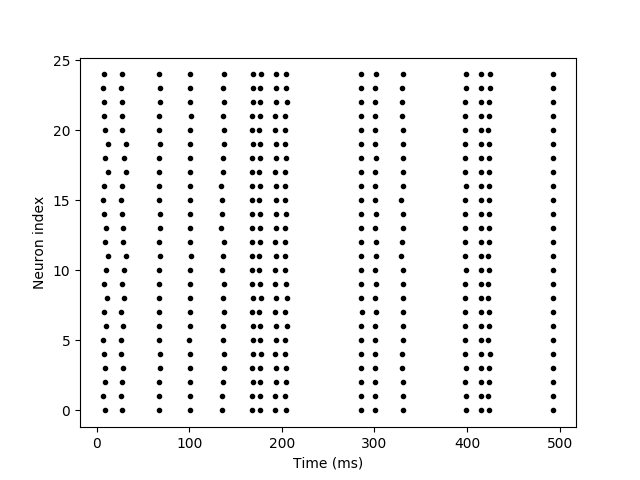# Example: reliability¶

Note

You can launch an interactive, editable version of this example without installing any local files using the Binder service (although note that at some times this may be slow or fail to open):Reliability of spike timing. See e.g. Mainen & Sejnowski (1995) for experimental results in vitro.

from brian2 import *

# The common noisy input
N = 25
tau_input = 5*ms
input = NeuronGroup(1, 'dx/dt = -x / tau_input + (2 /tau_input)**.5 * xi : 1')

# The noisy neurons receiving the same input
tau = 10*ms
sigma = .015
eqs_neurons = '''
dx/dt = (0.9 + .5 * I - x) / tau + sigma * (2 / tau)**.5 * xi : 1
'''
neurons = NeuronGroup(N, model=eqs_neurons, threshold='x > 1',
reset='x = 0', refractory=5*ms, method='euler')
neurons.x = 'rand()'
neurons.I = linked_var(input, 'x') # input.x is continuously fed into neurons.I
spikes = SpikeMonitor(neurons)

run(500*ms)
plt.plot(spikes.t/ms, spikes.i, '.k')
xlabel('Time (ms)')
ylabel('Neuron index')
show()